# Regular Polygons

## Overview

A polygon (from the Greek words "poly" meaning "many" and "gon" meaning "angle") is a closed, two dimensional figure with multiple (i.e. three or more) straight sides. A regular polygon is one in which all of the sides have the same length (i.e. the figure is equilateral) and all of the internal angles (and consequently all external angles) are of the same magnitude (i.e. the figure is equiangular). When we say that a figure is closed, we mean that exactly two sides meet at each vertex of the figure. The regular polygon may be simple or complex. A simple regular polygon is a convex figure in which the sides form a boundary around a single enclosed space, and no internal angle exceeds one hundred and eighty degrees. A complex regular polygon will be configured as a star, and the sides will intersect one another at various points. We present below some of the more commonly encountered convex regular polygons.

• Equilateral triangle - a three-sided figure with internal angles of sixty degrees (60°)An equilateral triangle

• Square - a four-sided figure in which all of the internal angles are right-angles (90°)A square

• Pentagon - a five-sided figure with internal angles of one hundred and eight degrees (108°)A pentagon

• Hexagon - a six-sided figure with internal angles of one hundred and twenty degrees (120°)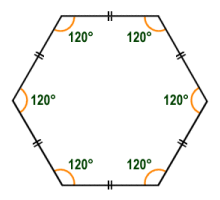A hexagon

As the number of sides increases, it is matched by the number of vertices. The magnitude of the internal angle at each vertex will be the same, and increases as the number of sides grows. The sum of the internal angles will simply be the internal angle at any vertex multiplied by the number of sides. If a polygon has n sides, the relationship between the number of sides and the internal angle at any vertex (in degrees) can be found using the following formula:

 Internal angle  =  (n  -  2)  × 180° n

Alternatively, we can express the internal angle in radians:

 Internal angle  =  (n  -  2)  × π n

The external angle at each vertex of a convex regular polygon will be the same, and will be the difference between the internal angle at that vertex and one hundred and eighty degrees, since the internal and external angles of a convex polygon are always supplementary (add up to one hundred and eighty degrees). The more sides a convex regular polygon has, the smaller the external angle at each vertex will be, because the internal angle will be larger. The sum of the external angles of a convex polygon will always be three hundred and sixty degrees (360°), so if a polygon has n sides, the relationship between the number of sides and the external angle at any vertex (in degrees) can be expressed using the following formula:

 External angle  = 360° n

We can also express the external angle in radians:

 External angle  = 2π radians n

The diagram below illustrates the relationship between the internal and external angles of a convex polygon. The figure shown here is a hexagon which means it has six sides, giving an internal angle of one hundred and twenty degrees (120°) and an external angle of sixty degrees (60°) at each vertex.Internal and external angles of a convex regular polygon are supplementary

We mentioned above that the sum of the internal angles can be calculated by multiplying the internal angle at any vertex by the number of sides. The following formula also works for a convex regular polygon with n sides, however, and does not require you to find the internal angle at a single vertex first:

Sum of internal angles  =  (n  -  2) × 180°

Details of other convex regular polygons are given in the table below. Note that in theory, there is no limit to the number of possible convex regular polygons, although as the number of sides and vertexes increases, the shape will start to look more and more like a circle.

Other Regular Polygons
ShapeNo. of SidesNameInt. AngleSum of Int. AnglesExt. Angle7Heptagon128.57° 900°51.43°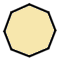8Octagon135.00°1080°45.00°9Nonagon140.00°1260°40.00°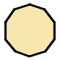10Decagon144.00°1440°36.00°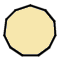11Hendecagon147.27°1620°32.73°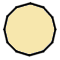12Dodecagon150.00°1800°30.00°

## The anatomy of a convex regular polygon

There is a point inside any convex regular polygon that is equidistant from the vertices of the polygon. This point, which is called the centre of the polygon, is also equidistant from the midpoints of the sides of the polygon. A line segment drawn from the centre to any vertex of a convex regular polygon is known as the radius of the polygon. It is also the radius of a circle that completely encloses the polygon and passes through all of the vertices. This circle is called the circumscribed circle or circumcircle. A second line segment, drawn from the centre to the midpoint of any side of a convex regular polygon is known as the apothem of the polygon. It too forms the radius of a circle, in this case one that is completely enclosed by the polygon and which touches each side of the polygon at its midpoint. This circle is known as the inscribed circle or incircle.The relationship between the radius (r), apothem (a), and side (s) of an octagonAn octagon with its circumcircle and incircle

You should be able to see from the above illustration that the size of the area of the octagon will fall somewhere between that of the incircle and that of the circumcircle. As the number of sides in a convex regular polygon increases, the polygon will become more and more like a circle, and the difference in length between the radius and apothem will become less and less significant. The areas of the polygon, incircle and circumcircle will likewise converge.

## Rotational symmetry

A line of symmetry drawn through a two-dimensional figure is one that divides the figure into two halves that are mirror images of one another. Regular polygons can have many lines of symmetry, allowing them to be rotated about their centre through some angle (other than three hundred and sixty degrees) without changing their appearance. The number of lines of symmetry is directly related to the number of sides a polygon has, so that an equilateral triangle has three lines of symmetry, a pentagon has five lines of symmetry, an octagon has eight lines of symmetry, and so on. The lines of symmetry for these shapes are illustrated below. Note that for regular polygons with an even number of sides and vertices, the lines of symmetry will pass through both pairs of opposite vertices and the midpoints of opposite sides. For regular polygons with an odd number of sides and vertices, each line of symmetry will pass through one vertex and the mid-point of the side opposite that vertex.A regular polygon with n sides has n lines of symmetry

## Diagonals

A diagonal in a regular polygon is any line segment other than a side that connects one vertex to another. Put another way, it is any line segment that connects two non-consecutive vertices. Any regular polygon with more than three sides has two or more diagonals. The number of diagonals increases with the number of sides n, and is related to n by the following formula:

 Number of diagonals  = n (n  -  3) 2

Let's examine the same regular polygons we used to demonstrate rotational symmetry, but this time with reference to their diagonals. The equilateral triangle has zero diagonals because the three line segments that join the vertices are all sides of the triangle. The pentagon has five sides and vertices and you can see from the illustration below that it also has five diagonals (using the above formula to find the number of diagonals for a pentagon will also yield a result of five). The last regular polygon shown here is the octagon, an eight-sided figure. You may well now start to experience some difficulty in counting the number of diagonals, but the formula tells us that there are in fact twenty (20). The squared term in the formula tells us that the number of diagonals increases exponentially as the number of sides increases.The number of diagonals increases exponentially with n

As well as the number of diagonals belonging to a particular regular polygon, mathematicians are also interested in the number of intersections between the diagonals (this includes the vertices of the polygon), the number of line segments that they cut each other into (this includes the sides of the polygon), and the number of regions (enclosed areas) that the intersections create within the polygon. Taking the pentagon as an example, we have ten (10) intersections, twenty (20) line segments, and eleven (11) regions. During the eighteenth century CE the Swiss mathematician Leonard Euler devised a formula relating the number of intersections, line segments and regions. Euler's formula is of particular interest in relation to the study of graph theory. Note, incidentally, that drawing all of the diagonals from one vertex only of a regular polygon will divide the polygon into n - 2 triangles, where n is the number of sides.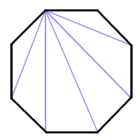The diagonals from a single vertex create n  -  2 triangles

## Finding the area of a convex regular polygon

Finding the perimeter of a regular polygon is a relatively trivial exercise. Once you know the length of one side, you simply multiply it by the number of sides to get the perimeter. Finding the area of a regular polygon is not quite so straightforward. The simplest regular polygon is the equilateral triangle. If we know the length of each side, we can find the area relatively easily, because the area of a triangle is found by multiplying the length of the base of the triangle by its height and dividing by two. If we take one side as the base of the triangle, we can use Pythagoras' theorem to find the height. Consider the following equilateral triangle: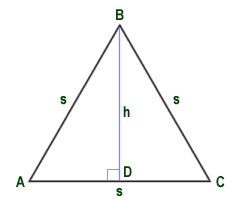Triangle ABC is an equilateral triangle

In equilateral triangle ABC, the length of each side of the triangle is represented by s. The height h of the triangle is the length of the perpendicular from the midpoint D of the base of the triangle to vertex B. Since triangle ABD is a right-angled triangle, Pythagoras' theorem tells us that the length of perpendicular BD, and hence the height h of the triangle, can be found using the following formula:

h  =  √(s2  -  (1/2s)2)

If we know the value of s therefore, we can find the height (BD) of the triangle and hence the area of the triangle (the area is equal to half the length of the base multiplied by the height). If we have a value for s of six (6), we get the following:

h  =  (62  -  32)  =  (36  -  9)  =  27  =  5.196

We can now calculate the area of the triangle:

 Area of triangle  = 6 × 5.196 =  15.588 2

Alternatively, we could use the following formula which allows us to find the area of an equilateral triangle without first having to find the height of the triangle, providing we know the length s of each side:

 Area of triangle  = s2 × √3 4

With this formula, if we again use a value for s of six, we get the following:

 Area of triangle  = 36 × 1.732 =  15.588 4

Finding the area of a square is a trivial exercise, since the area will simply be the length of one side multiplied by itself (i.e. squared). Finding the area of a regular convex polygon with more than four sides is not quite so straightforward although there is a simple formula that can be applied providing we know the length of each side and the length of the apothem (the line segment connects the centre of the polygon with the midpoint of any side). The length of the apothem for a given regular polygon with n sides of length s can be found using a number of different formulae, but since all of these formulae use trigonometric functions we will not discuss them here. Assume instead that for the purposes of this exercise we have found the apothem by measurement. We can use the following formula to find the area:

 Area of convex regular polygon  = ap 2

where:

• a  =  length of apothem
• p  =  length of perimeter

Consider the following octagon:The octagon has a side length of 6 and an apothem of 7.243

The octagon shown above has a side length of six (6), and an apothem of seven point two four three (7.243). The perimeter p of the octagon will be the length of one side (six) multiplied by the total number of sides (eight), which gives a value for p of forty-eight (48). We can now calculate the area of the octagon as follows:

 Area  = 7.243 × 48 =  173.832 2

In order to double check this result, we can approach the problem of finding the area of the octagon from a different perspective to see if we get the same result. Consider the following diagram, which shows the same octagon but with some additional construction:The octagon has internal angles of 135° and external angles of 45°

We already know that an octagon has internal angles of one hundred and thirty-five degrees (135°), and since the internal and external angles of a convex polygon are supplementary (i.e. they add up to one hundred and eighty degrees) then the external angles must be forty-five degrees (45°). triangle ABC therefore has two angles that are each forty-five degrees, so the remaining angle must be ninety degrees (90°) making this a right-angled triangle. Furthermore, with two angles having the same value, triangle ABC must be an isosceles right-angled triangle, which means that leg AB is the same length as leg BC. We can therefore use Pythagoras' theorem to find the length of line segments AB and BC:

 AB  =  BC  =  √ s2 =  √18  =  4.243 2

We can in fact construct four right-angled triangles as shown in the illustration below in order to convert the octagon into a square. The area of the square can be calculated by multiplying the total length of one side by itself (i.e. by squaring it).Four congruent triangles can be constructed to convert the octagon into a square

The area of the octagon can be found by subtracting the area of the four right-angled triangles from the area of the square. The calculation will be as follows:

 Area of octagon  =  (4.243 × 2 + 6)2  -  4  × 4.2432 2

Area of octagon  =  14.4862  -  4 × 9.001  =  209.844  -  36.004  =  173.84

The answer we get here is essentially the same answer we obtained previously, with the (very small) difference being due to rounding error. While this approach works for the pentagon, however, it does not work so well for other regular polygons, so the apothem formula is a more generally applicable solution. Let's see how it works.

The angle created at the centre of a convex regular polygon by two adjacent radius lines is known as the central angle. For an n-sided polygon, the central angle will be equal to three hundred and sixty degrees divided by n (360° ÷ n). The two radius lines, together with the side of the polygon to which they are mutually adjacent, will form an isosceles triangle. In the diagram below, one side s of a pentagon forms the base of isosceles triangle ABC, with the adjacent radii forming the triangle's lateral sides. Since apothem a forms the perpendicular that connects the base of the triangle with vertex B, then the height of the triangle will equal the length of the apothem.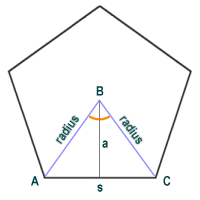An isosceles triangle is formed by one side and two adjacent radius lines

Each side of a polygon will form the base of an isosceles triangle, and the area of the polygon can be found by adding together the areas of all of the triangles thus formed. The area of a triangle is found using the following formula:

 Area of triangle  = base × height 2

The area of a polygon with n sides could thus be expressed as follows:

 Area of convex regular polygon  = n × base × height 2

We have already established that the length of the base of the triangle is equal to the length s of one of the sides of the polygon. We have also established that the length p of the perimeter of the polygon is equal to the number of sides n multiplied by the length s of each side. Given that the height of the triangle is equal to the length a of the apothem, we can re-write the formula as:

 Area of convex regular polygon  = ap 2

## Regular star polygons

Regular polygons that are not convex form various types of star shape, depending on the total number of vertices. As with a convex regular polygon, the vertices are equally spaced around some central point from which they are equidistant. Unlike a convex polygon, the sides do not connect the vertices in sequence. They may for example connect every other vertex, or every third vertex. In order to categorise these non-convex (star-shaped) regular polygons in a concise manner, we can use something called a Schäfli symbol. The Schläfli symbol is named after nineteenth century Swiss mathematician Ludwig Schläfli, and is used in geometry to denote the configuration of two and three-dimensional geometric shapes. In its most trivial form, it denotes the number of sides of a convex regular polygon, and takes the form {n} where n represents the number of sides. For example a pentagon, which is the simplest example of a convex regular polygon, would have the Schläfli symbol {5}.

Once we start looking at non-convex polygons the Schläfli symbol becomes slightly more useful, since it also tells us the "distance" (in terms of vertices) between two vertices that are connected by a single side of a polygon. Taking the pentagram as an example, the vertices are in exactly the same position that they would be for a pentagon, but the "sides" of the polygon connect alternate vertices. The Schläfli symbol for a pentagram would therefore be written as {5/2}, where the five represents the number of sides, and the two represents the distance between neighbouring vertices (i.e. vertices that are connected by a single line segment). Since we are talking about non-convex (essentially star-shaped) polygons, it might help to avoid confusion if you stopped thinking in terms of "sides", and instead thought in terms of "edges". The diagram below shows a pentagram. We have superimposed the pentagon that would be formed using the same vertices.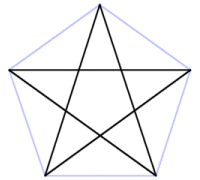A pentagram and a pentagon with a common set of vertices

The modified Schläfli symbol thus takes the form {n/m}, where n represents the number of sides (or edges) and m represents the distance between neighbouring vertices. The value of m represents, in approximate terms, the number of times the boundary of the polygon winds around the centre. The relationship between n and m determines another feature of the non-convex regular polygon, which is whether or not it will be degenerate. In order for the polygon to be non-degenerate, the values of n and m must be co-prime. In other words, the only integer that will divide them both to give a whole number result will be one. The pentagram, with values for n and m of five and two respectively, is non-degenerate (the only number by which both five and two can be divided to give a whole number result is one).

The edges of a pentagram cross each other at five points of intersection, which themselves form the vertices of a small internal pentagon. These intersection points are not counted as vertices, however, and the line segments that connect the intersection points to each other are not considered to be sides or edges in their own right. Interestingly, a pentagram can be created from a pentagon by extending the sides of the pentagon until they intersect. A larger pentagon can then be constructed by sequentially connecting the vertices of the newly-created pentagram. This process could in theory be repeated indefinitely to create ever larger pentagrams and pentagons. Here is a typical degenerate non-convex regular polygon, the octagram (Schäfli number {8/2}):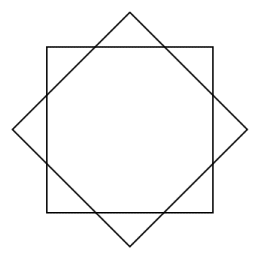An octagram has a Scläfli number of {8/2}

One tangible difference between a degenerate non-convex regular polygon and a non-degenerate one is that a non-degenerate non-convex regular polygon can be drawn by hand without lifting the pen (or pencil) from the paper, or going over the same line twice. In the field of computerised vector graphics, non-degeneracy is quite an important factor in determining the ease with which a figure can be rendered by a computer.